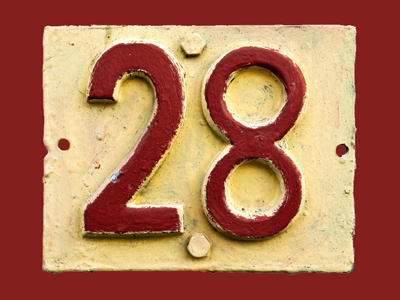28 is a multiple of 1, 2, 4, 7, 14 and 28.

# Multiples 2 (Very Easy)

Welcome to this, the second of our very easy Eleven Plus maths quizzes on multiples. By now you should know exactly what multiples are. If not, here’s a reminder:

A multiple is a number that may be divided by another a certain number of times without any remainder. So, 15 for example, is a multiple of 3 and 5 because it appears in both the three-times-table and the five-times-table. Likewise, 12 is a multiple of 2, 3, 4 and 6 because you will find the number twelve in all of those times tables.

If you are in any doubt, just ask yourself ‘does this number appear in that times table?’ If the answer is yes, then you’ve found yourself a multiple. Now. Let’s find out how well you can spot them. But take your time – carelessness costs marks in exams.

1.
Which of the four choices is a NOT multiple of ALL the numbers given?
2, 1, 7
14
42
35
28
The fact that 35 is an odd number tells you that it is not a multiple of 2
2.
Which of the four choices is a multiple of ALL the numbers given?
4, 5, 9, 10
40
50
90
180
40 isn't divisible by 9, 50 isn't divisible by 4 or 9, and 90 isn't divisible by 4
3.
Which of the four choices is a multiple of ALL the numbers given?
1, 13, 26
39
78
56
65
39 isn't divisible by 26, 56 isn't divisible by 13 or 26, and 65 isn't divisible by 26
4.
Which of the four choices is a multiple of ALL the numbers given?
45, 18, 10, 6
90
72
60
120
60 is not divisible by 45 or 18, 72 is not divisible by 10 or 45, and 120 is not divisible by 18 or 45
5.
Which of the four choices is NOT a multiple of ALL the numbers given?
1, 3, 7
42
21
63
14
14 is not divisible by 3
6.
Which of the four choices is a multiple of ALL the numbers given?
16, 4, 8, 2
28
24
32
40
28 isn't divisible by 8, 16 or 24, while 24 and 40 aren't divisible by 16
7.
Which of the four choices is a multiple of ALL the numbers given?
16, 10, 8, 40
40
160
120
60
40 and 120 aren't divisible by 16, while 60 isn't divisible by 8, 16 or 40
8.
Which of the four choices is NOT a multiple of ALL the numbers given?
1, 3, 9, 27
136
54
81
108
136 is not divisible by 3, 9 or 27
9.
Which of the four choices is NOT a multiple of ALL the numbers given?
2, 3, 6
102
78
144
99
99 is divisible by 1 and by 3, but not by 6
10.
Which of the four choices is NOT a multiple of ALL the numbers given?
24, 8, 6, 12
48
36
24
96
36 is divisible by 6, 8 and 12 but not by 24
Author:  Frank Evans